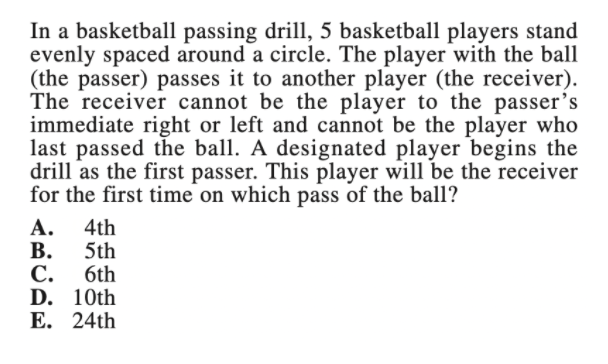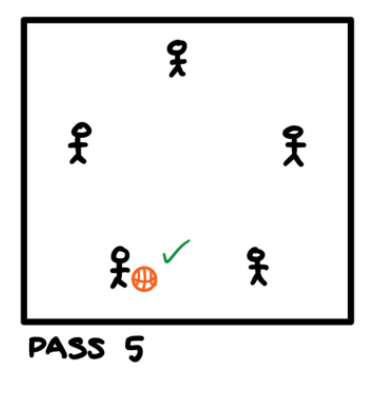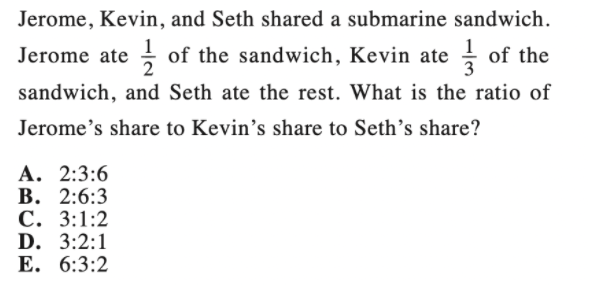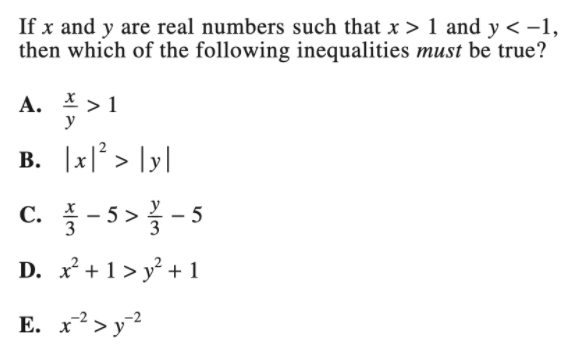Nisha Desai

# Guide to Pre-Algebra on the ACT Math Test + Practice Questions

### Do you know how to improve your profile for college applications?

See how your profile ranks among thousands of other students using CollegeVine. Calculate your chances at your dream schools and learn what areas you need to improve right now — it only takes 3 minutes and it's 100% free.

## What’s Covered:

Though pre-algebra is not listed as a specific subcategory on the ACT Math Test, almost every problem will involve some application of it. Thus, you’ll need to brush up on your pre-algebra knowledge as you study for the ACT Math test. In this post, we’ll go over some helpful practice problems so that you can test your pre-algebra skills.

## Overview of Pre-Algebra on the ACT Math Test

The ACT Math Test expects you to solve 60 multiple-choice questions in 60 minutes. A calculator is allowed during the entire test, but each question is designed so that a calculator is not necessary.

The types of questions each fall within the following five categories:

• Number & Quantity (7–10%)
• Algebra (12–15%)
• Functions (12–15%)
• Geometry (12–15%)
• Statistics & Probability (8–12%)

Though questions specific to pre-algebra only occur in about less than 10% of these questions, almost every question requires that you have strong knowledge of pre-algebra. For example, a geometry question might first involve knowing about angle congruence to set up the equations, then using pre-algebra to find the exact angle measure.

Not only will you be given an overall score for the ACT Math Test, but you will also receive subscores for each of the subsections in the Math test. The ACT also provides the following directions for the math section of the exam which we recommend you become familiar with prior to taking the exam:## How Will the ACT Impact My College Chances?

Your ACT Math Test will be scored out of 36. This is combined with your scores on the other sections of the ACT (English, Reading, and Science) to create a composite score out of 36. Your ACT composite score is used by colleges as a predictor of your collegiate academic success.

What this means is that your score will play an important role in your admissions chances (though this has changed recently due to COVID-19). To test how your score affects your chances, try out CollegeVine’s free chancing calculator. You can input your test scores, GPA, classes, and extracurriculars to get a more accurate prediction of your chances at the schools of your choice!## 10 Pre-Algebra Practice Questions

Here are a few practice questions (with solutions) so that you can test your pre-algebra content knowledge. Though some of them rely on other mathematical concepts, each of these questions requires a strong grasp of pre-algebra to successfully solve them.

### Question 1Let’s approach this problem by looking at each copy machine separately. First, let’s look at Machine 1. Machine 1 makes 60 copies per minute and makes copies for 8 minutes. So, the total number of copies made by Machine 1 is 60(8) = 480 copies.

Next, let’s look at the information given for Machine 2. If the second machine starts making copies 2 minutes after the first machine starts and stops making copies 8 minutes after the first machine starts, then the second copy machine operates for a total of 8 – 2 = 6 minutes. We also know that the second copy machine makes 80 copies per minute, giving us a total of 80(6) = 480 copies.

This gives us a total of 480 + 480 = 960 copies for both machines.

### Question 2The first step in this question is finding Marlon’s exact average. Let’s do this by taking the average of his five scores.

Mavg= (210 + 225 + 254 + 231 + 280)/5 = 1200/5 = 240

Remember that the ACT will not give you any formulas, so you’ll have to memorize how to compute an average. To maintain this exact average, the next score should also be 240. If this isn’t intuitive, we can also calculate what the next score should be to maintain an average of 240. We set up the following equation, with x representing the score for the 6th game:

[(Total of first 5 games) + x]/6 = 240

Since we know the total score of the first 5 games, we can solve for x:

(1200+x)/6 = 240

1200 + x = 240(6)

1200 + x = 1440

x = 240

### Question 3This question is one of the few that directly tests your pre-algebra skills. These questions may seem simple but are very easy to miss due to minor calculation errors.

If we start by dividing both sides by 9, we’ll have to deal with fractions. So, let’s instead start by distributing the 9:

9x – 81 = -11

9x = -11 + 81

9x = 70

x = 70/9

### Question 4This question first involves translating verbal information into equations, then using pre-algebra to solve. Let’s extract information from the question one sentence at a time.

First, if the discount tickets sell for \$4.00 each, and Enrico bought d tickets, the total cost of the discount tickets is 4d.

Next, it says that Enrico spent \$60.00 on discount tickets. This means we can create the following equation: 4d = 60.

This means that d = 15, so Enrico bought 15 discount tickets.

Next, we are told that this cost is \$37.50 less than if the tickets were bought at the regular price. So, the total cost of the tickets that were bought at the regular price is 37.50 + 60 = \$97.50. If the price of regular tickets is p, and we know that 15 tickets were bought, we have that 15t = 97.50. We can now solve for t, the regular ticket price.

t = 97.50/15 = 6.50

### Question 5Once again, this question involves a more direct application of pre-algebra. For questions like these, it’s important to pay close attention to your work and keep track of your exponents and arithmetic.

Let’s solve this by writing out each step carefully to reduce the chances of making mistakes. We can use the FOIL method, which involves multiplying the following pairs:

• First terms
• Outside terms
• Inside terms
• Last terms

So, in our case we have: (3x)(3x) + (3x)(4y2) + (-4y2)(3x) + (-4y2)(4y2) = 9x2 + 12xy2 – 12xy2 – 16y4 = 9x2 – 16y4

Alternatively, we can recognize that this expression is of a special form. When we have an expression of the form (a – b)(a + b), we know the following equation:

(a – b)(a + b) = a2 b2

This is called the Difference of Two Squares (DOTS) method. Remember, the ACT doesn’t give formulas, so you’d have to have this prior knowledge. But, knowing this makes solving the problem much quicker since we can merely square both terms and take the difference:

(3x)2 – (4y2) = 9x2 – 16y4

## Discover your chances at hundreds of schools

Our free chancing engine takes into account your history, background, test scores, and extracurricular activities to show you your real chances of admission—and how to improve them.

### Question 6Like many other ACT math questions, we’ll need to convert verbal information to numeric equations. If we have two consecutive integers, and we let the first integer be x, we have that the second integer is x + 1.

If we add the smaller integer plus triple the larger integer, we get x + 3(x + 1).

This simplifies to: x + 3x + 3 = 4x + 3.

We know that this sum is equal to 79, so we have the following equation:

4x + 3 = 79

4x = 76

x = 19

Then, x + 1 = 19 + 1 = 20. So, our two integers are 19 and 20.

### Question 7To better visualize this scenario, let’s draw a picture. We can keep drawing pictures for each pass until we find a pattern or reach a point where the ball is passed back to the first player.If Player A has the ball, they’re only able to pass to Players C or D (since they can’t pass to a player to the immediate right or left).

Let’s arbitrarily assume that player A picks player C for the first pass:Now, Player C can’t pass to Players B or D (since they’re to the immediate left and right) and Player C also can’t pass to Player A (since Player A last passed the ball). So, their only option is to pass to player E:Now, Player E can’t pass to the players to the immediate left and right (Players A and D), and the last player who passed the ball (Player C), so they will then pass the ball to Player B:Again, we see that the only option for Player B to pass to is Player D:Now, with the same logic, Player D must pass to Player A:With this fifth pass, Player A once again has the ball, so the answer to this question is B.

### Question 8Though this question requires knowledge of statistics, you’ll need pre-algebra to successfully complete the problem.

If we want the probability of randomly drawing a red marble to be ⅗, we need the proportion of red marbles to be equal to ⅗.

So, if we let r represent the number of additional red marbles, the total number of marbles becomes 32 + r and the total number of red marbles becomes 12 + r.

Then, the proportion of red marbles is ( 12 + r)/(32 + r). Let’s set this equal to ⅗ and use pre-algebra to solve.

(12 + r)/(32 + r) = 35

We cross multiply:

3(32 + r) = 5(12 + r)

96 + 3r = 60 + 5r

2r = 36

r = 18

### Question 9Before we determine the ratio, let’s start by figuring out Seth’s share of the sandwich. If Jerome ate ½ and Kevin ate ⅓ of the sandwich, Seth was left with 1 – ½ – ⅓.

We can solve by using a common denominator of 6:

6/6 3/6 2/6 = 1/6

Now, we have the following portions:

Jerome: ½

Kevin: ⅓

Seth: ⅙

An easy way to determine the ratio is to again change all the fractions to a common denominator:

Jerome: 3/6

Kevin: 2/6

Seth: 1/6

So, the ratio is 3:2:1.

### Question 10We can rely on the guess and check method to easily solve this problem. Let’s first pick arbitrary values of x and y to eliminate some answer choices.

Let’s consider the case where |x| and let x = 3 and y = -10.

Because x/y = -3/10 < 1, answer choice A is incorrect.

Because |x|2 = (3)2 = 9 and |-10| = 10, we have that |x|2 < y, so answer choice B is also incorrect.

Because x2 + 1= (3)2 + 1 = 10 and y2 + 1 = (10)2 + 1 = 101, we have that x2 + 1 < y2 + 1, so answer choice D is also incorrect.

Now, let’s consider the case where |x|>|y| and let x = 3 and y = -2.

Then, x-2 = 1/x2 = 1/9 and y-2 = 1/y2 = 1/4. This means that x-2 < y-2 , so answer choice E is also incorrect.

This means that answer choice C is correct. We can manipulate the inequality to see why this is true: (x/3 – 5) > (y/3 – 5)

We add 5 to both sides: x/3 > y/3

We multiply both sides by 3: x > y.

We know this is true since we are given that x > 1 > -1 > y, which simplifies to x > y.

## Final Tips and Strategies

Rather than focus on pre-algebra specifically, you can build your pre-algebra skills while solving other questions on the ACT. Since many geometry, statistics, and functions questions implicitly test your pre-algebra skills, as you answer those questions, try to notice what parts are more difficult.

If the geometric or statistical concepts are harder, focus your studying on learning those concepts. But, if the calculations are more tricky, you may need to brush up on your pre-algebra skills. As you take practice tests and complete sample problems, pay close attention to your calculations and make sure you’re taking the time to double-check your work.

### Memorize formulas

Since the ACT doesn’t provide any formulas, having basic formulas memorized will be key to successfully completing the exam. We’ve determined the important formulas that you’ll need to know, so you can start by tackling this list. Having formulas memorized often simplifies problems that might otherwise be more complicated.

In fact, certain questions (like those that involve area or volume, for example) will require that you know specific formulas. Practicing problems involving formulas is also a good way of putting your pre-algebra skills to the test. In many cases, once you’ve correctly identified which formula to use, it’s just a matter of implementing pre-algebra to solve for the correct answer.

Take a look at some of CollegeVine’s other guides to the ACT Math Test:

#### Want more college admissions tips?